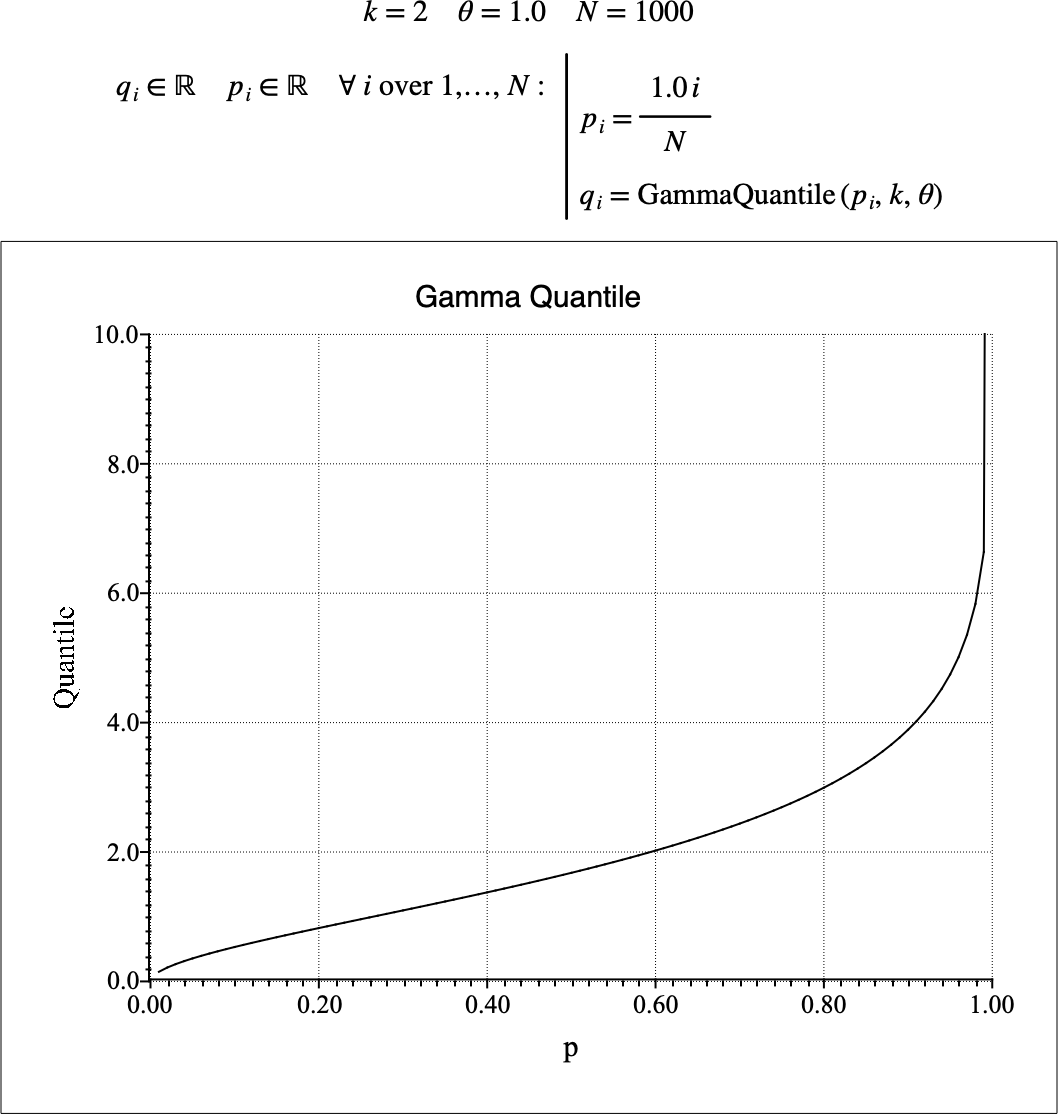# $$\text{GammaQuantile}$$¶

You can use the $$\text{GammaQuantile}$$ function to calculate the quantile function of the gamma distribution. The quantile function is the inverse of the cumulative distribution function.

You can use the \gammaq backslash command to insert this function.

The following variants of this function are available:

• $$\text{real } \text{GammaQuantile} \left ( \text{<p>}, \text{k} \right )$$

• $$\text{real } \text{GammaQuantile} \left ( \text{<p>}, \text{k}, \text{<}\theta\text{>} \right )$$

Where $$p$$, $$k$$, and $$\theta$$ are scalar values representing the cumulative probability, the shape term, and the scale term respectively. Note that this function is defined over the range $$0 \leq p \leq 1$$, $$k > 0$$, and $$\theta > 0$$ and will generate an error or return NaN for values for which the function is not defined.

The $$\theta$$ term is optional. The value 1 will be used if the $$\theta$$ term is not provided.

The value is calculated directly using the relation:

$\text{GammaQuantile} \left ( p, k, \theta \right ) = \gamma\text{Inv} \left [ k, p \Gamma \left ( k \right ) \right ] \theta$

Where $$\gamma\text{Inv} \left ( s, z \right )$$ is the inverse lower incomplete gamma function.

The gamma distribution can be viewed as the sum of $$k$$ identical and independent exponential distributions, each with rate $$\frac{1}{\theta}$$. The exponential distribution is therefore the special case of the gamma distribution with $$k = 1$$. Similarly, the gamma distribution is also equivalent to the Erlang distribution when $$k$$ is an integer value.

The gamma distribution is identical to the chi-squared distribution with $$v$$ degrees of freedom when $$k = \frac{1}{2} v$$ and $$\theta = 2$$.

Figure 143 shows the basic use of the $$\text{GammaQuantile}$$ function.Figure 143 Example Use Of the GammaQuantile Function